# SSC Reasoning Ability Practice Questions (Day-57)

Dear Aspirants, The SSC Exams are going on at national level. Millions of candidates have eagerly prepared for the ongoing SSC exams such as CGL, CHSL, MTS, CPO, JE etc. SSC exams are usually conducted by the Staff Selection Commission to recruit the eligible candidates for various Central government departments. Mostly the selection process for all the SSC exams consists of the single or double phase of written examination based on the examination. Due to that, the written exam consisted of a certain syllabus and exam pattern. By following those regulations, the written exam has been conducted through an online mode. Based on the latest updated exam pattern and syllabus, here we have drafted the Reasoning Ability questions on a daily basis. So aspirants start your practice session with us and mould your skill in a perfect shape.

Start Quiz

Direction (1): In the following question, select the number which can be placed at the sign of question mark (?) from the given alterantives.

1)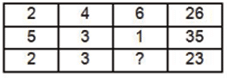(a) 7

(b) 8

(c) 9

(d) 10

Directions (2-3): How many quadrilaterals are there in the given figure?

2)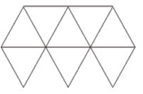(a) 14

(b) 15

(c) 17

(d) 19

3) How many triangles are there in the given figure ?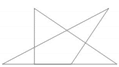(a) 5

(b) 7

(c) 8

(d) 10

Direction (4-5): In each of the following question below are given some statements followed by some conclusions. Taking the given statements to be true even if they seem to be at variance from commonly known facts, read all the conclusions and then decide which of the given conclusion logically follows the given statements.

4) Statements:

I) All boys are smart.

II) All smart are thin.

Conclusions:

I) All boys are thin.

II) All smart are boys.

(a) Only conclusion (I) follows

(b) Only conclusion (II) follows

(c) Both conclusion follow

(d) Neither conclusion (I) nor conclusion (II) follows

5) Statements:

I) All cups are pencils.

II) Some pencils are pens.

Conclusions:

I) Some pencils are cups.

II) No pencil are cups.

III) Some cups are pens.

(a) Only conclusion (I) follows

(b) Only conclusion (III) follows

(c) Only conclusion (I) and (II) follow

(d) Only conclusion (II) and (III) follow

6) From the given options, which answer figure can be formed by folding the figure given in the question?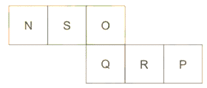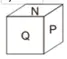(a)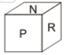(b)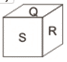(c)(d)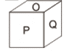7) Two position of a cube are shown below. What will come opposite to face containing ‘B’?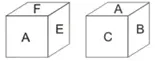(a) C

(b) E

(c) F

(d) A

8) In the given figure, how many wooden plates are there?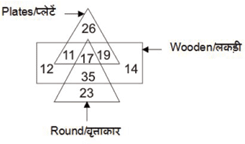(a) 30

(b) 47

(c) 36

(d) 28

9) In the given figure, how many sour fruits are there?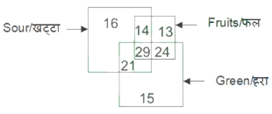(a) 27

(b) 53

(c) 50

(d) 43

10) In the given figure, how many moving cars are not red?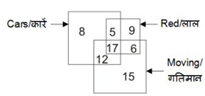(a) 22

(b) 17

(c) 12

(d) 29

22 + 42 + 6 = 26

52 + 32 + 1 = 35

22 + 32 + 10 = 23

7 triangles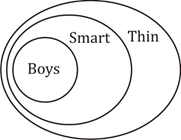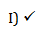II) X

∴ Only conclusion I follows.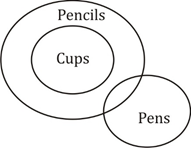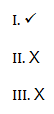Only conclusion I follows.

Sol. Opposite faces are

N ↔ O

Q ↔ P

S ↔ R

∴ Option (b) can be formed.

F is opposite  to B

Wooden plates = 11 + 17 + 19 = 47

Sour fruits = 14 + 29 = 43